• 此文档以一个小的实例讲解了java随机数random的使用，在java当中随机数的使用率较高，所以需要熟练掌握随机数的使用
• 本程序利用C++产生随机数，每次产生的随机数都不一样
• random.random() 生成一个0-1的随机浮点数 import random random.random() random.uniform(a,b) 生成一个指定范围内的随机浮点数. ...从指定范围内，按指定基数递增的集合中获取一个随机数random..
 random.random() 生成一个0-1的随机浮点数 import random
random.random()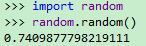random.uniform(a,b) 生成一个指定范围内的随机浮点数. random.uniform(1, 10)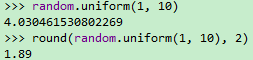random.randint(a,b) 生成一个指定范围内的整数 random.randint(3, 7)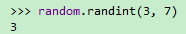random.randrange(start, stop, step) 从指定范围内，按指定基数递增的集合中获取一个随机数。 random.randrange(2, 10, 3)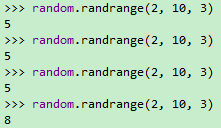random.choice(sequence) 从sequence（不是一种特定的类型，而是泛指一系列的类型，list， tuple，字符串等都属于sequence）表示的有序类型中，选择一个值。 random.choice('random function study!')
random.choice(['random', 'function', 'study'])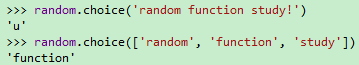random.shuffle(x) 用于将一个列表中的元素打乱 x = ['random', 'function', 'study']
random.shuffle(x)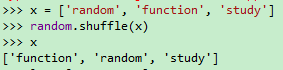random.sample(sequence, k) 从指定序列中随机获取指定长度的片段，sample函数不会修改原有序列。 ls = [1, 3, 5, 7]
random.sample(ls, 2)
ls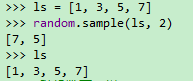random.seed() 随机数生成的种子，当seed没有参数时，每次生成的随机数是不一样的，而当seed有相同参数时，每次生成的随机数是一样的，选择不同的参数生成的随机数也不一样。 #随机数不一样
random.seed()
random.random()
random.seed()
random.random()
#随机数一样
random.seed(1)
random.random()
random.seed(1)
random.random()
random.seed(2)
ranodm.random()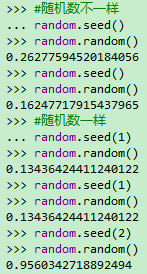展开全文python
• java.util.Random是Java中一个可以生成随机数的类，并且你可以通过传入相关参数来对随机数的范围进行控制，无论在实际开发中，还是学习生活中，Random随机数都能起到比较大的作用。那么今天就来学习一下Random的使用...
java.util.Random是Java中一个可以生成随机数的类，并且你可以通过传入相关参数来对随机数的范围进行控制，无论在实际开发中，还是学习生活中，Random随机数都能起到比较大的作用。那么今天就来学习一下Random的使用！
相信你肯定抽过奖！那么作为一个程序员，你有没有想过那些抽奖的大转盘，或者是九宫格是怎么实现的呢？又是怎么控制中奖概率的呢？跟着我的脚步，我带你领略Random的风骚！
实例化对象
通过源码可以看到Random类是有两个构造方法的，其中一种是不带参的，还有一种是带有一个long类型参数（称为：种子）的，那么这两种有何区别呢？
不带参：完全随机
带参：传入参数（种子）的Random对象所生成的随机数是固定的
举个栗子，当你实例化两个Random对象时，如果参数（种子）都传入10，那么这两个Random（random1 和 random2）对象所生成的随机数，random1生成的第一个随机数 = random2生成的第一个随机数 ，random1生成的第二个随机数 = random2生成的第二个随机数 ，代码如下：
        //实例化Random对象
Random random = new Random();
Random random2 = new Random();

Random random3 = new Random(10);
Random random4 = new Random(10);

for (int i = 0; i < 10; i++) {
int number=random.nextInt();
int number2=random2.nextInt();
int number3=random3.nextInt();
int number4=random4.nextInt();
//输出格式是：  无种子随机数  无种子随机数  有种子随机数  有种子随机数
System.out.println(number+"_"+number2+"___"+number3+"_"+number4);
}

输出结果：
1075217332 -2135067742 -1157793070 -1157793070 -944171219 -837885942 1913984760 1913984760 1314450533 1413088185 1107254586 1107254586 215653709 -337612687 1773446580 1773446580 1737734575 1766714434 254270492 254270492
从输出的五组数来看，可以明显地发现，无种子随机数生成的随机数是完全随机的（没有任何联系），而传入了种子的两个random对象所生成的随机数是一样的，得出结论：传入种子的Random对象所生成的随机数是固定的
注意：种子不会影响随机数的范围
常用方法
Random的几个常用方法都比较简单，这里只列举三种最常用的。
生成 int 类型随机数
这里演示获取多种类型的 int 数据的方法：默认范围 [int最小值,int最大值) 、0~最大范围 [0,max) 、自定义范围 [min , max) 、自定义范围 [min , max] ，当然了这里只演示几种最常用的，还有很多其他类型的，都可以通过不同的公示来进行改变
//生成默认范围（int类型的范围）的随机数
int i = random.nextInt();//[int最小值,int最大值)

//生成自定义 0~最大范围（max）的正整数随机数 [0,max)
int i = random.nextInt(max);//[0,10)

//生成自定义范围随机数 [min , max)
//公式：  .nextInt(max) % (max - min) + min
int i=random.nextInt(10)%(10-5)+5;//[5,10)

//生成自定义范围随机数 [min , max]
//公式：  .nextInt(max) % (max - min + 1) + min
int i = random.nextInt(10) % (10 - 5 + 1) + 5;//[5,10]

生成 boolean 类型随机数
这个很好理解，就是直接生成 true 和 false 中的一个，概率相同
boolean b = random.nextBoolean();

生成 double 类型随机数
nextDouble()生成的随机数范围是 [0 , 1.0) 的小数
double d = random.nextDouble();

随机数的几种常用用途
随机数在很多地方都用得到，我个人最常见的就是应用在抽奖中了，一个抽奖程序，想要控制各种奖项的概率，用随机数来做再合适不过了！
中奖概率的控制：
中奖概率控制原理：通过获取随机数，然后利用 if 语句先限定中奖的区间，就可以达到一个公平的概率效果！
        //首先在 [1,100] 区间（100个数）随机获取一个数
int i = random.nextInt(100) % (100 - 1 + 1) + 1;
System.out.println("当前获取的随机数：" + i);
//然后根据中间概率来设置 if 条件语句的区间，达到效果
if (i >= 80) {// [80,100] 一等奖
System.out.println("恭喜，一等奖！");
} else if (i >= 50) { // [50,80] 二等奖
System.out.println("恭喜，二等奖！");
} else {//[1，50]  谢谢惠顾
System.out.println("很遗憾，没有中奖！");
}

骰子的制作：
        //首先在 [1,6] 区间（6个数）随机获取一个数
int i = random.nextInt(6) % (6 - 1 + 1) + 1;
System.out.println("骰子点数：" + i);

硬币正反面也是同理，这里面随机数的区间以及中奖的概率都可以根据实际需要来做调整！
展开全文java android
• ## java中Random随机数使用

千次阅读 多人点赞 2020-04-26 17:08:15
Random 使用步骤： 1.导包 import java.util.Random; 2.创建对象 Random r = new Random(); 3.获取随机数 int number = r.nextInt(10); //获取数据的范围：[0,10)包括0，不包括10 例子：获取1到100之间的随机数 /...
Random
使用步骤：
1.导包
import java.util.Random;

2.创建对象
Random r = new Random();

3.获取随机数
int number = r.nextInt(10);
//获取数据的范围：[0,10)包括0，不包括10

例子：获取1到100之间的随机数
//获取1到100之间的随机数
//创建对象
Random r = new Random();

for (int j = 0; j < 10; j++) {
//获取随机数
int i = r.nextInt(100);//当前范围是0~99
i += 1;//现在范围就是1~100
System.out.println(i);
}


猜数字小游戏案例
​ 系统产生一个1到100之间的随机数，请猜出这个数据是多少。
分析：
​ 1.系统产生一个1到100之间的随机数
​ 2.从键盘获取用户输入数据
​ 3.比较这两个数据的，用if语句实现：
​ 大了：提示大了！
​ 小了：提示小了！
​ 猜中了：提示恭喜你，猜中了！
​ 4.多次猜数据，利用while(true)进行多次循环，猜中用break结束循环
public static void main(String[] args) {
//生成1到100之间的随机数
Random r = new Random();
int num = r.nextInt(100) + 1;
//从键盘获取用户输入信息
Scanner scanner = new Scanner(System.in);

while (true){
System.out.println("请输入一个1~100之间的数字：");
int n = scanner.nextInt();
if (n >= 1 && n <= 100){
if (n > num){
System.out.println("大了！");
}else if (n < num){
System.out.println("小了！");
}else {
System.out.println("恭喜你，猜对了！");
break;//猜对循环结束
}
}else {
System.out.println("输入不合法！");
}
}

scanner.close();
}

展开全文java
• 主要为大家详细介绍了Java常用工具类，Random随机数工具类、MD5加密工具类，具有一定的参考价值，感兴趣的小伙伴们可以参考一下
• ## C# Random随机数

千次阅读 2019-01-09 21:33:23
1 Random类简介 &amp;amp;amp;amp;amp;amp;amp;nbsp; &amp;amp;amp;amp;amp;amp;amp;nbsp; &amp;amp;amp;amp;amp;amp;amp;nbsp; &amp;amp;amp;amp;amp;amp;amp;nbsp; Random 类 命名空间：System ...
转载至：点击这里
1 继承关系
Object→Random 表示伪随机数生成器，这是一种能够产生满足某些随机性统计需求的数字序列的设备。
2 Random类简介
Random 类 命名空间：System 表示伪随机数生成器，一种能够产生满足某些随机性统计要求的数字序列的设备。 伪随机数是以相同的概率从一组有限的数字中选取的。所选数字并不具有完全的随机性，因为它们是用一种确定的数学算法选择的，但是从实用的角度而言，其随机程度已足够了。
3 Random成员
序号成员名描述1Equals确定指定的 Object 是否等于当前的 Object。（继承自 Object。）2Finalize允许 Object 在“垃圾回收”回收 Object 之前尝试释放资源并执行其他清理操作。（继承自 Object。）3GetHashCode用作特定类型的哈希函数。（继承自 Object。）4GetType获取当前实例的 Type。（继承自 Object。）5MemberwiseClone创建当前 Object 的浅表副本。（继承自 Object。）6Next已重载。 返回随机数。7NextBytes用随机数填充指定字节数组的元素。8NextDouble返回一个介于 0.0 和 1.0 之间的随机数。9Sample返回一个介于 0.0 和 1.0 之间的随机数。9ToString返回表示当前 Object 的 String。（继承自 Object。）
4 Random生成随机数示实例
4.1生成可重复的随机数
生成小于100的非随机数
static void Main(string[] args)
{
Random rand = new Random();
for (int i = 0; i < 100; i++)
{
Console.WriteLine(rand.Next(100));
}
}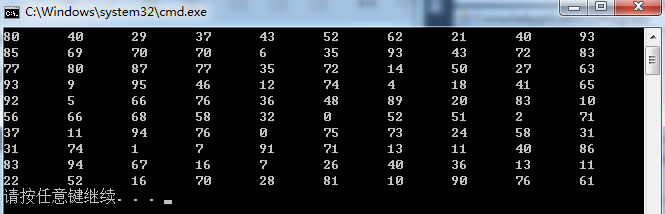生成某区间的随机数
static void Main(string[] args)
{
Random rand = new Random();
for (int i = 0; i < 100; i++)
{
Console.WriteLine(rand.Next(5,10));
}
}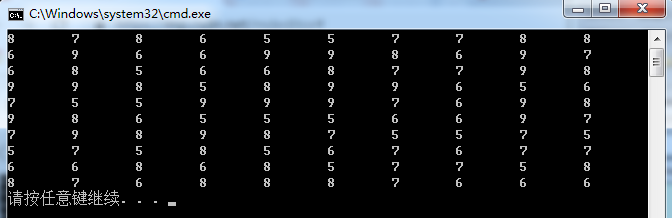生成0.0-1.0间的随机数
static void Main(string[] args)
{
Random rand = new Random();
for (int i = 0; i < 100; i++)
{
Console.WriteLine(rand.NextDouble());
}
}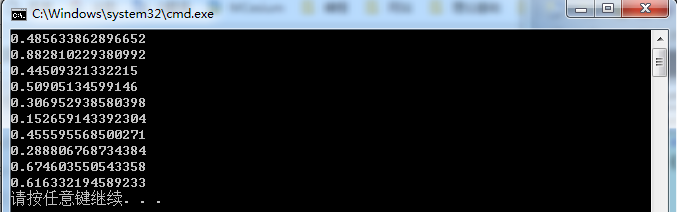4.2生成不重复的随机数
下面使用两种方法生成不重复随机数，并计算耗时。  ①使用Hashtable（using System.Collections;）
//生成不重复的
static void Main(string[] args)
{
//设置计时起始时间
DateTime beforDT = System.DateTime.Now;
Hashtable hashtable = new Hashtable();
Random rm = new Random();
int RmNum = 10000;
for (int i = 0; hashtable.Count < RmNum; i++)
{
int nValue = rm.Next(20000);
if (!hashtable.ContainsValue(nValue))
{
//Console.Write(nValue + "\t");
}
}
DateTime afterDT = System.DateTime.Now;
TimeSpan ts = afterDT.Subtract(beforDT);
Console.WriteLine("DateTime总共花费{0}ms.", TotalMilliseconds);
}②使用条件判断
//生成不重复的
static void Main(string[] args)
{
DateTime beforDT = System.DateTime.Now;
Random rand = new Random();
int j = 1;
int[] result = new int;
result = rand.Next(20000);
for (int i = 0; j < 10000; i++)
{
int temp = rand.Next(20000);
for (int r = 0; r < j; r++)
{
if (result[r] == temp)
{
break;
}
if (r == j - 1)
{
result[j] = temp;
j++;
}
}
}
DateTime afterDT = System.DateTime.Now;
TimeSpan ts = afterDT.Subtract(beforDT);
Console.WriteLine("DateTime总共花费{0}ms.", ts.TotalMilliseconds);
}从上面计算耗时来看，Hashtable用时比普通算法要多出3倍。
展开全文• Random生成的随机数都是伪随机数！！！ 是由可确定的函数（常用线性同余），通过一个种子（常用时钟），产生的伪随机数。这意味着：如果知道了种子，或者已经产生的随机数，都可能获得接下来随机数序列的信息（可...Java nanoTime
• 当“随机数种子”相同的时候，每次调用该函数产生的结果都一样，所以在实际运行环境中，我们应该避免使用相同的随机数种子，常用的方法就是使用System.nanoTime来为作为种子。java
• 1、利用RANDOM随机数 shell有一个环境变量RANDOM,范围是0--32767 如果我们想要产生0-25范围内的数：\$((\$RANDOM%26)) 在$(()) 是可以省略取值的$符号的。（All tokens in the expression undergo parameter ...shell
• ## 破解 RANDOM随机数

千次阅读 2019-04-07 13:56:30
下列字符串 efbaf275cd、4be9c40b8b、44b2395c46、f8c8873ce0、b902c16c8b、ad865d2f63 是通过对随机数变量 RANDOM随机执行命令： echo $RANDOM|md5sum|cut –c1-10 后的结果，请破解这些字符串对应的RANDOM值 ... • 解决了C# Random生成随机数重复问题,并生成随机数的 介绍了五种方法。 没有分的童鞋，可以参考博文，博文里有源码： http://blog.csdn.net/shuai_wy/article/details/78606175 • Math,random随机数的 各个位获取 相加Math • int random=(int)(Math.random()*90+10); //提示用户输入两位数 java.util.Scanner input=new java.util.Scanner(System.in); System.out.println("请输入两位数的随机数"); int num = inp • 程序生成一个随机数，指示它是否大于或小于0.5 程式码范例 运行random.js 动机 没有任何动力 安装 仅下载 API参考 它没有API 测验 节点ramdom.js。 贡献者 何塞·曼努埃尔·伯恩（Jose Manuel Burned） 执照 麻省...JavaScript • 就是Random类的简单随机数产生···就是这样，给需要的人！ • 答：random模块1. 生成一个0-1之间的随机数：（不包括1） x = random.random() 例如： x = 0.52365212582. 生成一个指定范围内的浮点数： x = random.uniform(10, 20) 例如： x = 18.12345874... • Random.nextInt(int n),此方法就是取大于等于0，小于n的随机整形数数。 Math.random()*int n,此方法取得随机数同上方法一样 • 目录 import numpy as np ...2、np.random.normal(loc, scale, size) 3、np.random.uniform(low, high, size) 4、np.random 5、numpy.random.RandomState() import numpy as np 1、np.li...Numpy Python3 数据分析 • ## IDEA-Random随机数 千次阅读 2021-02-15 13:40:25 Random类用来生成随机数字 1.导包 import java.util.Random; 2.创建 Random r = new Random();//小括号当中留空即可 3.使用 获取一个随机的int数字（范围是int所有范围，有正负两种） ：int num = r.nextInt() 获取... • 可以使用System.currentTimeMillis()，但这这种方式获取的不能称为严格意义的随机数，虽然他是一直变的，但他不是随机的。表示获取当前系统时间，产生一个当前的毫秒，这个毫秒其实就是自1970年1月1日0时起的毫秒数 ...java • 产生1个n~m范围内的int型随机数: random.randint(n,m) random.randint(1,5) 产生1个n~m之间的float型随机数: random.uniform(n, m) random.uniform(n, m) 产生1个0~1之间的float型随机数: random.random() ...python numpy • Java中生成随机数的方式 很多时候我们需要一些随机数，尤其是做测试的时候。 在Java中生成随机数有几种方式 Java.util.UUID-生成唯一的字符串 可以通过UUID类生成唯一的字符串，并且是全局...Math.random() 通过Mat... • 猜字游戏 random 随机数 变色 真聪明 太笨了 • ## Linux RANDOM随机数 万次阅读 2012-08-08 09:22:49 在BASH环境下,这个RANDOM变量的内容是得到0~32767之间的随机数. 所以你只要echo$RANDOM时,就会主动的随机取出一个介于0~32767之间的数值. 如果我们想要0~100之间的呢.我们就可以利用 declarelinux bash c
•python
• .net平台自带的一个Random类是非常基础的，需要实例化才能使用，不可避免的产生GC，在unity engine里面也封装了一个，全部都是静态的方法，不需要new直接使用，而且针对unity环境添加了很多的数值类型的随机数。...
• Math是JavaScript的一个对象方法，... 以下方法是分别生成0-n以内的随机数 和x-y以内的随机数。 求0-n 以内的随机数， 包括0和n Math.round(Math.random()*n) //0-10 console.log(Math.round(Math.random()...JavaScript
• Python中的random模块用于生成随机数。下面介绍一下random模块中最常用的几个函数。 random.randomrandom.random()用于生成一个0到1的随机符点数: 0 random.uniform　random.uniform的函数原型为：random.uniform(a...python list...